# Stress Plot PropertyManager

The Stress Plot PropertyManager allows you to plot stress results for static, nonlinear, dynamic, and drop test studies.

To display this PropertyManager, run a static, nonlinear, dynamic study, or drop test study. Right-click Results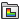and select Define Stress Plot.

## Display

Solid and Shell Available only for mixed mesh studies with beams. Plots stress components for solids or shells only.
Beams Available only for mixed mesh studies with beams. Plots stress components for beam only.
Render beam profile (slower) Available only for studies with beams. Displays stress plot on actual beam geometry as opposed to cylinders. See Beam Stress Components.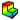Component Select a stress component to plot. Directions are based on the selected reference geometry. See Stress Components.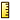Units Select the units of the stress plot.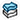Shell Face Select one of the following:
 Top Total stresses (bending + membrane) at the top face. For composite shell, Top refers to top face of selected ply in Ply number under Composite Options. Bottom Total stresses (bending + membrane) at the bottom face. For composite shell, Bottom refers to bottom face of selected ply in Ply number under Composite Options. Membrane Membrane stress component Bending Bending stress component.
Beam Results Select one of the following:
 Axial Uniform axial stress Upper bound bending in DIR 1 Highest magnitude of bending stress due to moment about local direction 1, M1. Upper bound bending in DIR 2 Highest magnitude of bending stress due to moment about local direction 2, M2. Upper bound axial and bending Highest stresses at the extreme fibers of the cross-section. Combined uniform axial stress and the two bending stresses due to M1 and M2. Torsional Highest magnitude of torsional stress (shear stress due to torque). Shear in DIR 1 Highest magnitude of shear stress due to shear force in local direction 1. Shear in DIR 2 Highest magnitude of shear stress due to shear force in local direction 2.
Beams or trusses under axial compressive loads show a negative axial stress value and beams under axial tensile loads show a positive axial stress value.
Click Settings > Show beam directions to view the local beam direction Dir 1 and Dir 2.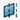Plane, Axis or Coordinate System Select a reference plane, an axis, or a coordinate system to plot stress. Used for directional components only. Show as vector plot Generates a vector plot where a vector is plotted at each node to show the magnitude and direction of the selected component. You can control the size and density of the vectors in a vector plot using the Vector Plot Options PropertyManager. Available for directional stress components only. Show as tensor plot Available only for von Mises stress (VON). The three principal stresses at nodes or at element centers are shown. Render the thickness for shells (slower) Displays the stress results on shell bodies using a 3D representation of the shells. Stress results at the top and bottom shell faces are shown. Results are linearly interpolated across the shell thickness. The shell body is displayed with the thickness value defined in the Shell Definition PropertyManager. The shell thickness orientation is displayed with relation to the midsurface of the shell as defined by the offset value in the Shell Definition PropertyManager. Show plot only on selected entities Generates plots only for the selected entities.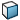Select faces for the plot Select the faces to view results.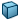Select bodies for the plot Select the bodies to view results. Display symmetric results Available for models with symmetry restraints or cyclic symmetry. Select to view the stress values for the whole model. The results are mirrored about the planes of symmetry for models where you analyze half, a quarter, or one-eighth of the actual geometry. For cyclic symmetry, results are repeated cyclically about the defined axis of revolution. Node Values Generates nodal stress plots. Linear interpolation is used to generate a smooth plot. Element Values Generates stresses at centers of elements (one value/color for each element). Average results across boundary for parts Averages results at the common nodes between two components in the assembly.

## Plot Step

Available only for nonlinear, dynamic and drop test studies.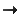Plot for Single Step Select this option to plot the results at the selected solution step.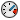Sets the time (in seconds) at which the stress results are plotted. If there are no results available for the requested time, results are plotted for the nearest available solution step number.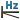Available for harmonic and random vibration studies. Displays the frequency corresponding to the selected plot step.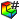Plot Step Sets the solution step number for the plot.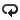Plot Bounds across All Steps Select this option to plot extreme values regardless of the solution step they occur at. Select one of the following:
 Maximum Displays the maximum algebraic values (envelope plot) across all solution steps. Minimum Displays the minimum algebraic values (envelope plot) across all solution steps. Absolute maximum Displays the absolute maximum values (envelope plot) across all solution steps.

## Deformed Shape

If checked, the selected component is plotted on the deformed shape of the model.

 Automatic Displays the default Scale Factor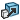that the program uses to scale the largest deformation to 10% of the largest dimension of the smallest box that surrounds the model. True scale Displays the actual deformed shape of the model (Scale Factor is 1.0). User defined Allows you to enter your own Scale Factor. In most cases, a larger scale factor helps to appreciate the deflection.

## Composite Options

Available only for composite shells.

 Maximum across all plies Displays the maximum stress values of the specified component (envelope plot) across all plies. The program includes the Top and Bottom faces while searching for the extreme value across all plies.Ply number Select the ply number to show the stress. This option is not available when you select Maximum across all plies. Display results in ply direction on composite surfaces (Available only when SX: X Normal Stress is the selected stress component). Shows stress along the ply direction on surface of the ply. Display results in cross-ply direction on composite surfaces (Available only when SY: Y Normal Stress is the selected stress component). Shows stress in the transverse direction to the ply direction on surface of the ply.

## Property

 Include title text Type a custom title for the plot. Associate plot with name view orientation Associate a predefined view orientation with the active plot.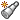Associate with current view Associates the current view with the active plot.